Type a math problemalgebra
trigonometry
statistics
calculus
matrices
variables
list
AC
log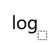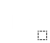ln
(
)
7
8
9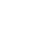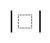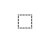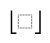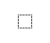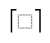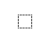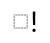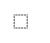τ
π
4
5
6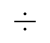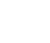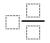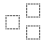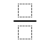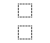%
θ
1
2
3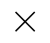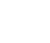<
>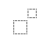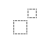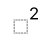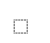x
i
0
.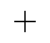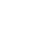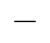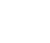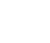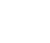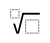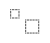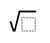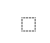y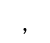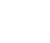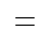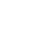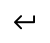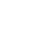EvaluateSteps Using Derivative Rule for QuotientFor any two differentiable functions, the derivative of the quotient of two functions is the denominator times the derivative of the numerator minus the numerator times the derivative of the denominator, all divided by the denominator squared.
The derivative of a polynomial is the sum of the derivatives of its terms. The derivative of a constant term is . The derivative of is .
Do the arithmetic.
Expand using distributive property.
To multiply powers of the same base, add their exponents.
Do the arithmetic.
Remove unnecessary parentheses.
Combine like terms.
Subtract from .
For any term , .
For any term except , .
View solution steps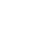Graph

## Similar Problems from Web Search

\frac{\left(x^{1}-5\right)\frac{\mathrm{d}}{\mathrm{d}x}(3x^{2}-2)-\left(3x^{2}-2\right)\frac{\mathrm{d}}{\mathrm{d}x}(x^{1}-5)}{\left(x^{1}-5\right)^{2}}
For any two differentiable functions, the derivative of the quotient of two functions is the denominator times the derivative of the numerator minus the numerator times the derivative of the denominator, all divided by the denominator squared.
\frac{\left(x^{1}-5\right)\times 2\times 3x^{\left(2-1\right)}-\left(3x^{2}-2\right)x^{\left(1-1\right)}}{\left(x^{1}-5\right)^{2}}
The derivative of a polynomial is the sum of the derivatives of its terms. The derivative of a constant term is 0. The derivative of ax^{n} is nax^{\left(n-1\right)}.
\frac{\left(x^{1}-5\right)\times 6x^{1}-\left(3x^{2}-2\right)x^{0}}{\left(x^{1}-5\right)^{2}}
Do the arithmetic.
\frac{x^{1}\times 6x^{1}-5\times 6x^{1}-\left(3x^{2}x^{0}-2x^{0}\right)}{\left(x^{1}-5\right)^{2}}
Expand using distributive property.
\frac{6x^{\left(1+1\right)}-5\times 6x^{1}-\left(3x^{2}-2x^{0}\right)}{\left(x^{1}-5\right)^{2}}
To multiply powers of the same base, add their exponents.
\frac{6x^{2}-30x^{1}-\left(3x^{2}-2x^{0}\right)}{\left(x^{1}-5\right)^{2}}
Do the arithmetic.
\frac{6x^{2}-30x^{1}-3x^{2}-\left(-2x^{0}\right)}{\left(x^{1}-5\right)^{2}}
Remove unnecessary parentheses.
\frac{\left(6-3\right)x^{2}-30x^{1}-\left(-2x^{0}\right)}{\left(x^{1}-5\right)^{2}}
Combine like terms.
\frac{3x^{2}-30x^{1}-\left(-2x^{0}\right)}{\left(x^{1}-5\right)^{2}}
Subtract 3 from 6.
\frac{3x^{2}-30x-\left(-2x^{0}\right)}{\left(x-5\right)^{2}}
For any term t, t^{1}=t.
\frac{3x^{2}-30x-\left(-2\right)}{\left(x-5\right)^{2}}
For any term t except 0, t^{0}=1.